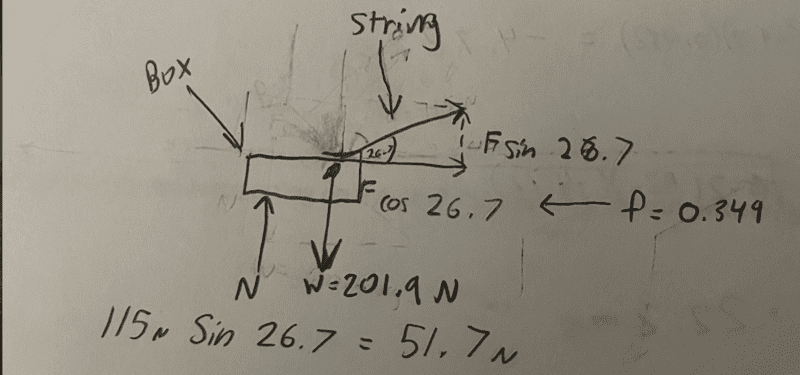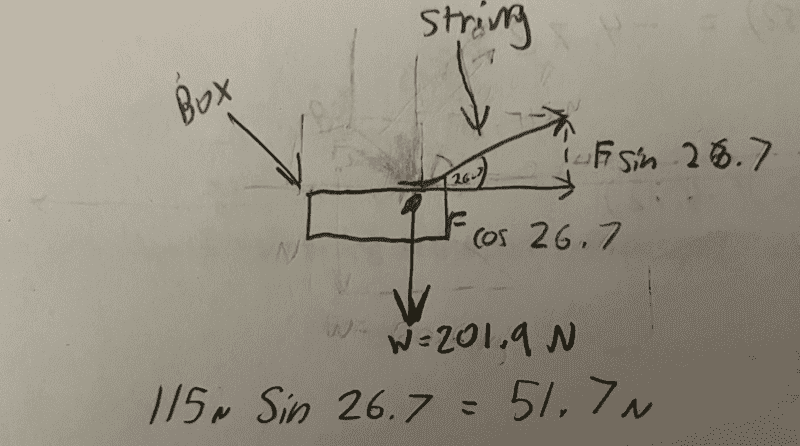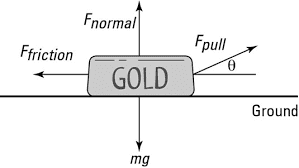# Pulling a crate across the floor with a rope at an angle

• jevans123
I see. You are asking for the normal force of the floor pushing up on the crate.Sorry, I misunderstood. I thought you were asking for the normal force of the floor pushing up on the crate. In that case, the expression would be:Ff=μkN

#### jevans123

Homework Statement
I was told to be specific, so here it is:

Roy pulls up at an angle on a rope attached to a crate to move the crate across a horizontal floor. The mass of the crate is 20.6 kilograms, Roy applies 115 newtons of force at an angle of 26.7 degrees, and the coefficient of kinetic friction between the crate and the floor is 0.349.

a. How hard does the floor push up on the crate?

b. What is the acceleration of the crate?
Relevant Equations
f = mu x N
I drew out a motion diagram and used 115N sin(26.7 degrees) = 51.7 N but It turns out to be wrong.Last edited:
jevans123 said:
Homework Statement:: Roy pulls up at an angle on a rope attached to a crate to move the crate across a horizontal floor. The mass of the crate is 20.6 kilograms, Roy applies 115 Newtons of force at an angle of 26.7 degrees, and the coefficient of kinetic friction between the crate and the floor is 0.349.

a. How hard does the floor push up on the crate?

b. What is the acceleration of the crate?
Relevant Equations:: f = mu x N

I drew out a motion diagram and used 115N sin(26.7 degrees) = 51.7 N but It turns out to be wrong.
You will have to be more specific than that in your attempts. You are not giving any reasoning or even stating what you are trying to compute.

••topsquark and jevans123
I'm sorry, what does that even mean? Why are people just unwilling to help? Like I genuinely am asking a question and everybody just does some weird homework template and ohhh I haven't given enough detail and all. Like I am just asking for help and if you can't help then what is the point of commenting what you commented? I'm new so it would be nice to explain but I'm just so confused right now. What does "simultaneity" even mean and how is that even relevant?!

jevans123 said:
What does "simultaneity" even mean and how is that even relevant?!
The word "simultaneity" appears in the signature that @Orodruin uses. It has nothing whatsoever to do with the response he provided.
jevans123 said:
a. How hard does the floor push up on the crate?
[...]
jevans123 said:
I drew out a motion diagram and used 115N sin(26.7 degrees) = 51.7 N but It turns out to be wrong.
Responding to the only work that was shown...

You have correctly calculated the vertical component of the tension force on the crate. Is that the quantity that was requested for part a?

•topsquark
jbriggs444 said:
The word "simultaneity" appears in the signature that @Orodruin uses. It has nothing whatsoever to do with the response he provided.

[...]

Responding to the only work that was shown...

You have correctly calculated the vertical component of the tension force on the crate. Is that the quantity that was requested for part a?
Well, the reason why I calculated the vertical component was because it was asking for how hard does the floor push up on the crate which is essentially the normal force and the term "up" tells me to find the vertical component. I don't think that is all to it but its all that I have gotten and understood.

jevans123 said:
Well, the reason why I calculated the vertical component was because it was asking for how hard does the floor push up on the crate which is essentially the normal force and the term "up" tells me to find the vertical component. I don't think that is all to it but its all that I have gotten and understood.
You are asked for the force of the floor on the crate.
You found the force of the rope on the crate.
Are those the same thing?

Have you drawn a free body diagram?
Can you make a list of the forces that act on the crate?

•topsquark
List:

so we have,

the box being pulled at an angle of 26.7 degrees at 115 Newtons of force

then we have the weight of the crate going directly downward, Oh wait you made me think. Mass is not the same as weight. So now I'm thinking I use the mass of the box which is 20.6kg and multiply that by the Earth's constant acceleration of 9.80 and now the weight is 201.9 Newtons directly downward.

then we have the coefficient of kinetic friction directed the opposite of the way of the box at 0.349

jbriggs444 said:
Have you drawn a free body diagram?
jevans123 said:
the box being pulled at an angle of 26.7 degrees at 115 Newtons of force
Please show us this (your FBD) before we go much farther. You can upload a PDF of JPEG copy of your diagram by using the "Attach files" link below the Edit window. Thank you.

•topsquarkYou don't show all forces acting on the block. The surface is also exerting a normal force and a force of kinetic friction.It is customary to draw the forces acting on the body as arrows with their tail on the body. See example below. Also, the force of friction is not 0.349. That number is the coefficient of kinetic friction. What is the expression that allows you to find the force of friction if you know the coefficient of kinetic friction?•berkeman and topsquark
OHHH ok so it would be this:

Ff=μkFN

But you need normal force to find that and I don't have normal force yet. Is normal force just the equal to the weight (in Newtons) of the object

No. You need to add all the forces that have vertical components and set the sum equal to zero because the acceleration is zero in that direction.

•topsquark
jevans123 said:
OHHH ok so it would be this:

Ff=μkFN

But you need normal force to find that and I don't have normal force yet. Is normal force just the equal to the weight (in Newtons) of the object
You seem to be missing a major concept in this problem. Newton's 2nd Law says that net force acting on an object is equal to its mass times its acceleration. ie. ##F_{net} = ma##. Force and acceleration are vectors so this is a vector law. So we have the net force component in the horizontal direction and the net force component in the vertical direction.

We know from the problem statement that the acceleration component in the vertical (call it y) direction is 0 because we are not lifting the box. We also know (or need to calculate) an acceleration in the horizontal (call it x) direction. So we have
##\sum F_x =ma##

##\sum F_y = 0##

Break each of your forces into components and plug them into the Newton's 2nd Law equations.

-Dan

•jevans123